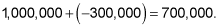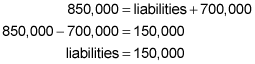##### Accounting: 1,001 Practice Problems For DummiesYou need to be careful when using the accounting equation because there are two versions of the formula. One is assets – liabilities = equity. The other is assets = liabilities + equity. Keep in mind which version you’re using.

Here are a couple of practice questions to help you get familiar with this formula.

## Practice questions

1. At the end of an accounting period, a company’s total assets equaled \$576,000, and liabilities equaled \$245,000. How much was the company’s owners’ equity?

2. The owners of a start�?up invest \$1,000,000 into the business. After one year of operations, the business has assets of \$850,000 and losses of \$300,000. What are the total liabilities at the end of the first year?

1. \$331,000

The basic accounting equation is assets = liabilities + owners’ equity.

You can always double-check your answer by going back to the original equation assets = liabilities + owners’ equity. In this example, the sum of liabilities of \$245,000 and owners’ equity of \$331,000 is \$576,000. This corresponds to the given amount of total assets, so you know your answer is right.

2. \$150,000

You need to go back to the basic accounting equation: assets = liabilities + owners’ equity. Step one is to determine total owners’ equity, which has two parts — the investment by owners and the losses that the business experienced during the first year of operations. Investment by owners is \$1,000,000. So, the total owners’ equity is \$700,000:Now you can use the basic accounting equation to calculate total liabilities at the end of the year:If you need more practice on this and other topics from your accounting course, visit Dummies.com to purchase Accounting For Dummies! Featuring the latest information on accounting methods and standards, the information in Accounting For Dummies is valuable for anyone studying or working in the fields of accounting or finance.